# Top 50 interview questions of Machine Learning

By Manas Kochar Category Machine Learning Reading time 33-42 mins Published on May 27, 2021

Crack The Top MNC Jobs by Answering the Most Asked Interview Questions of Machine Learning

Machine Learning is one of the most astounding technological advancements in the modern era. This recent development and the popularity of machine learning algorithms have greatly changed the organization's focus on data-driven decision-making. As a result, the job vacancies in machine learning are at their peak. But as the number of vacancies is increasing and skill criteria, as well as the competition, are also getting high. This makes cracking a machine-learning interview is becoming a tough job.

Here are the top 50 trending interview questions of machine learning, along with their most appealing answers. This will surely help you if you are going to face an MNC machine learning interview in 2023.

## 50 Useful Machine Learning Interview Questions You Should Know

Typically, machine learning interview questions have appeared in a variety of domains. This blog deepens into the machine learning interview questions to make getting the information you need easier.

### 1. What are Overfitting and Underfitting in Machine Learning?

Ans: Two main problems occurring in machine learning that degrade the ML model's performance are Overfitting and Underfitting.

Overfitting occurs mainly in supervised learning. When a machine learning model tries to cover more data points than required in a dataset, the model begins caching noise and incorrect values in the dataset. This decreases the model's accuracy and efficiency.

Underfitting occurs because of overfitting in a model. The training data being fed may be stopped due to overfitting, thereby leaving insufficient training data to learn from. The ML model may not discover the best match for the prevailing trend in the data. This reduces accuracy and results in irregular outcomes.

### 2. How to handle categorical variables in KNN?

Ans: By generating dummy variables from categorical variables and using them rather than the actual category variable, they can be handled. Don't create (k-1) instead of k dummy variables.

Let's take "Age Group" as a categorical variable name that has 5 distinct categories/levels. Therefore, we generate 5 dummy variables. '1' as a value is allocated to every dummy variable that belongs to a department, and in another case, '0' is allocated as a value.

### 3. Is KNN suitable for regression? How to apply KNN to Regression?

Ans: Yes, the K-nearest neighbor (KNN) is suitable for regression. We can employ the KNN algorithm when a continuous dependent variable is present. Taking the mean of the values from k to its closest neighbors will give us the predicted value.

### 4. Compare KNN and K Means Algorithms.

Ans: In contrast to K-means, a form of unsupervised machine learning, KNN would be supervised machine learning. While a regression or classification ML algorithm is employed by KNN, K-means employs a clustering ML algorithm. When every data presents the same scale, this situation is suitable for KNN but not for K-means.

### 5. Besides altering k how can the model's higher variance be reduced?

Ans: If the number of samples drawn from the original dataset is not limited, a sample variance reduction strategy would be to sample numerous times and then use the major votes in the KNN models to fit each of these samples and categorize all the test data points. This method, known as bagging, is a way of reducing variation.

### 6. How does sampling affect KNN?

Ans: Because KNN works in such a point-by-point manner, sampling accomplishes numerous tasks from the viewpoint of a single data point.

• As the dataset gets scarce, the average distance toward the k-nearest neighbors rises.

• As a result, the region covered by k-nearest neighbors grows in size, thus covering a bigger portion of the feature space.

• And sample variance increases.

The rise in variance is a result of such a change in input. Variance refers to the difference seen in projections made from various population samples. Why might the immediate impacts of sampling raise the model's variance?

Notice how the same k data points now represent a greater region of the feature space. The population space it depicts has grown even while the sample size has not grown. As a result, the proportion of classes within k closest data points will have a bigger variance, as will the categorization of each data point.

### 7. What will happen if we change the K's value in KNN?

Ans: As k rises, the class limits of the predictions get more smooth.

Lowering the k value renders the KNN model more "sensitive." In other words, it has a higher sensitivity to local variations in the dataset. The model's "sensitivity" immediately corresponds to its variance.

The examples demonstrate the inverse connection between variance and k. Consider what happens to KNN when k attains its maximum value, k=n (n refers to points present inside the practice set). In this scenario, the projections are led by the major class present in the practice set. It will select the most prevalent class inside the data and never stray, resulting in virtually zero variance. As a result, to minimize variance, k should be raised.

Final Verdict: To counterbalance the increased variance caused by sampling, k can be adjusted to reduce model variance.

### 8. What is the thumb rule for approaching the KNN problem?

Ans:

Step 2: Initialize the value of k

• Find the distance between each training data row and the test data. Because it is the most often used approach, we shall utilize Euclidean distance as our distance metric. Chebyshev, cosine, and other metrics can also be employed.

• Sort the computed distances by distance value in ascending order.

• Get the first k rows of the sorted array.

• Identify the most prominent class of these rows.

• After receiving the projected class, return it and repeat the process up to the amount of training information points that were received.

KNN Code Snippet: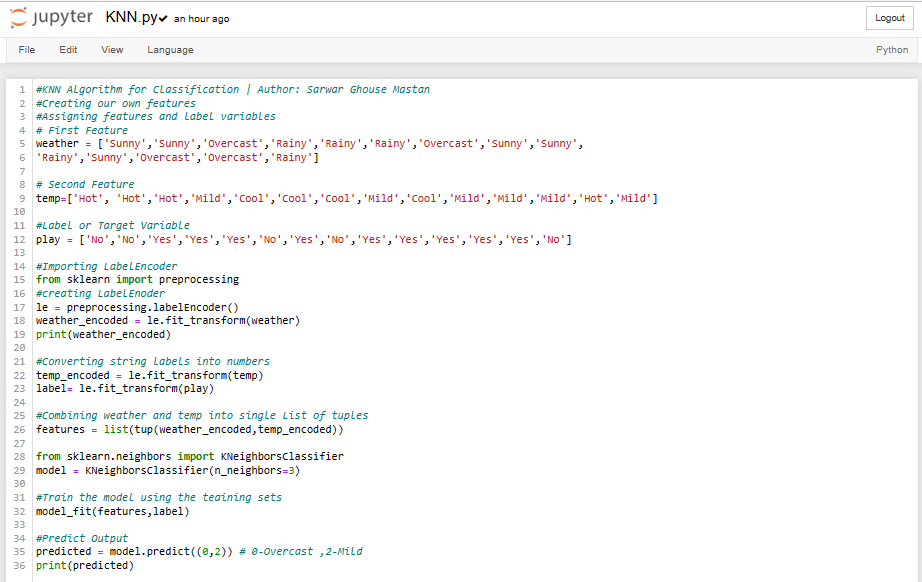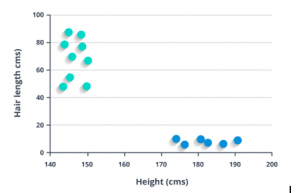Now we'll look for some lines dividing the data into two distinct data groups. Along this line, each group's closest points will have the most distance.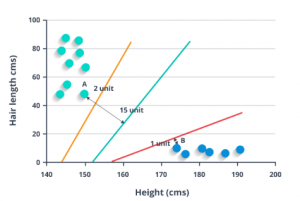The line dividing the dataset into two distinctly categorized groups is known as the classifier line because the two closest endpoints are at the farthest separation from the line. The new data may then be classified on the basis of where on each side of the line the testing data fits.

### 9. What is an SVM Algorithm?

Ans: Machine Learning is one of the most astounding technological advancements in the modern era. This recent development and the popularity of machine learning algorithms have greatly changed the organization's focus on data-driven decision-making. As a result, the job vacancies in machine learning are at their peak. But as the number of vacancies is increasing and skill criteria, as well as the competition, are also getting high. This makes cracking a machine-learning interview is becoming a tough job.

### 10. Explain Support Vectors.

Ans: The line having the largest difference within 2 classes, called the "best" line, is found using Support Vector Machines. The points on this margin are called Support Vectors.

### 11. What purpose does a Support Vector serve in SVM?

Ans: An SVM classifies data by locating the hyperplane that optimizes the separation gap within two classes. In the data sets composing the hyperplane, support vectors are the largest spots.

### 12. What do you mean by kernels?

Ans: Kernels are a group of mathematical operations that SVM algorithms rely on. The kernel's function changes the received input info into a suitable form. Various sorts of kernel functions are employed by several SVM algorithms. There are four different kernel types in SVM.

• Polynomial kernel
• Linear Kernel
• Sigmoid kernel

### 13. What is meant by Kernel Trick?

Ans:
It enables us to interact in the initial feature space without having to compute data coordinates in a greater space.

1. SVMs find the n-1 higher dimensional space to separate a dataset having n features (n-dimensional).

2. Non-linearly distinct sets of data are not suitable for SVMs.

3. Currently, SVM can handle these datasets.

4. However, it is frequently feasible to turn our non-linearly separable dataset into a higher-dimensional dataset that is linearly separable, allowing SVMs to perform well.

5. Unfortunately, the number of dimensions you must add (through transformations) frequently relies on how many dimensions you currently have (and not linearly).
It becomes practically challenging for datasets with several characteristics to test out all of the interesting modifications.

6. Here comes the Kernel Trick.

7. Fortunately, SVMs only need to compute the pair-wise dot products in the (higher-dimensional) feature space (during training).

8. The kernel function is presented as aimed at a specific vector set in a low-dimensional subspace. Also, a conversion into a high-dimensional space is possible. The dot product can be analyzed without explicitly transforming the vectors inside the high-dimensional space.

9. We are saved!

### 14. Why is SVM called a Large Margin Classifier?

Ans:
It positions the decision border so that the separation between the two clusters is maximized.

The hyperplane where the gap from the data sets is maximum should be chosen, which the geometric margin formalizes. This is how it's characterized: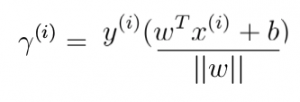It is just the standardized functional margin. As a result, the maximum margin classifier-a forerunner of the SVM-was created.

### 15. What are the variations in SVM and Logistics Regression?

Ans:

• SVM seeks the best gap between the support vectors and the line that divides the classes, lowering the chance of data inaccuracy, whereas Logistic Regression does not. Instead, it has many decision limits with varying weights that are close to the optimum point.
• SVM works best with semi-structured and unstructured data, such as text and pictures, whereas Logistic Regression fits with independent variables that have previously been defined.
• SVM is based on data geometry, whereas Logistic Regression is dependent on statistical techniques.
• SVM may be used to analyze sets of data not separable linearly, but Logistic Regression cannot.
• Overfitting occurs in Logistic Regression but is less likely in SVM.

### 16. When Should Logistic Regression be used over Support Vector Machine?

Ans: You can use a support vector machine or logistic regression depending on the number of training datasets/features you have.

As an example, consider the following:

n is the number of features, while m denotes the number of training samples.

• n is large and m is small: SVM alongside a linear kernel/Logistic Regression.
• n is small and m is moderate: SVM using a kernel (eg. Polynomial, Gaussian, etc.).
• n is a small number, m is a huge number: Use SVM alongside a linear kernel/Logistic Regression, after linking more characteristics yourself.

### 17. What does the C and Gamma parameter in SVM signify?

Ans:

The high energy boundaries determining how well an SVM model operates are gamma and cost. An ML model must carefully balance its bias and variance.

High Gamma values for SVM provide greater precision but produce false outcomes, and vice versa. Similarly, a high-cost parameter (C) value suggests poor accuracy but less bias, and vice versa.

Choose a model with the least bias and variance. As a result, you must select the appropriate values for C and Gamma.

Using approaches such as Grid search, optimal C values and Gamma may be identified.

By how much the training examples should be stopped from being incorrectly classified by the SVM operator can be referred to by the C parameter. If the optimizer correctly classifies each of the training points for high C values, it may choose a smaller-margin hyperplane. A rather small C value will cause the optimizer to seek a larger gap between the hyperplane and the points, even though the hyperplane misclassifies numerous points. Misclassified cases should be expected for very small values of C, even if your training data can be separated linearly.

The gamma parameter characterizes the impact of one training sample, typically low values indicating "far" and large values indicating "near."

The gamma parameters may be considered the opposite of the radius of effect of samples chosen as support vectors by the model. The impact sphere's area comprises the support vector itself when Gamma is extremely big. Overfitting can't be stopped using C regularization in this case.

When Gamma is very tiny, the model is too limited and cannot describe the data's complexity or "shape." The whole training set would be included in the zone of influence of any chosen support vector. The resultant model will operate in the same way as a linear model containing a series of hyperplanes separating any two classes' high-density centers.

### 18. Discuss SVM's plus points and downsides.

Ans:

• When we don't know anything about the data, SVMs come in handy.
• Even unstructured & semi-structured data such as text, images, & trees work well.
• SVM's kernel trick seems to be a real strength. We can solve any difficult issue with a suitable kernel function.
• SVM, unlike neural networks, does not solve for local optima.
• It scales reasonably well to high-dimensional data sets.
• In reality, SVM models have generality; the danger of over-fitting is lower in SVM.
• The SVM has always been compared to the ANN. In regards to performance, SVMs beat ANN models.

• It is difficult to select a "good" kernel function.
• Long training time for large datasets.
• The individual impact, variable weights, and final model are difficult to grasp and analyze.
• We can't conduct modest calibrations to the model since the final model isn't easily visible, making it difficult to include our business logic.
• Gamma and Cost C are the high energy boundaries of SVM. These hyper-parameters are difficult to fine-tune. It is difficult to imagine their influence.

SVM code snippet: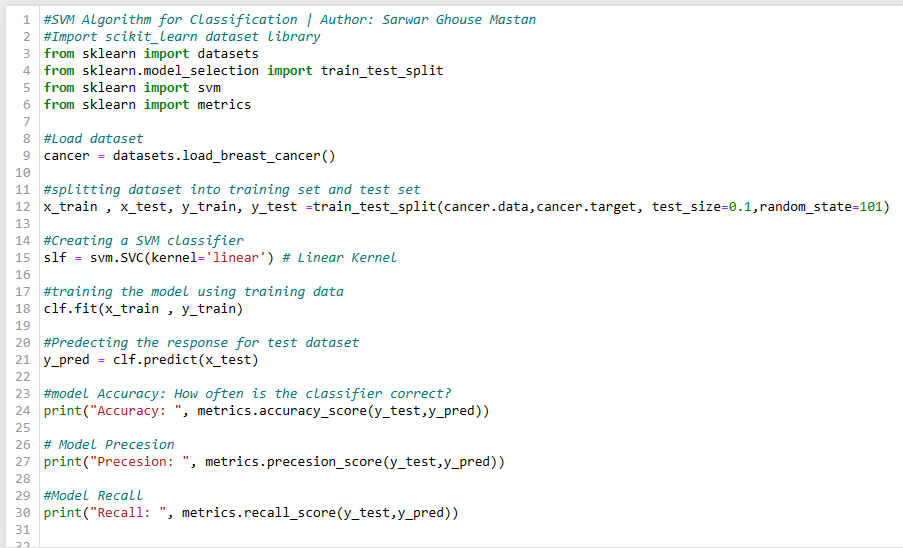### 19. What is the Reinforcement Learning technique?

Ans: Reinforcement Learning is a response-based Machine Learning technique in which an agent understands how to act in a specific environment by performing actions and watching the results. In general, a reinforcement learning agent can detect and grasp its environment, act, and improve via trial and error. Positive feedback is given to the agent for each positive activity; negative feedback or a penalty is given to the agent for each negative behavior.

### 20. What is Naïve Bayes Algorithm?

Ans: It is a classification method that estimates the likelihood of each data point relating to a group and afterward classifies the point as belonging to the category with the greatest likelihood.

Discussion on Bayes' Theorem.

By combining the conditional probability provided a result and prior knowledge of an event occurring, Bayes' Theorem calculates the likelihood of an event occurring.

The chance that an event will occur, given that a certain event occurred, is known as conditional probability. Conditional probability, or P(X|Test), is the possibility of X for a test outcome. For instance, what is the likelihood that an email is a spam if my spam filter flagged it as spam?

The previous likelihood is determined using preceding knowledge or the percentage of prior samples. For instance, what is the likelihood that any email is a spam?

Formally

• Posterior probability = P(A|B) = The likelihood that A occurred if B occurred.
• Conditional probability = P(B|A) = The likelihood of B occurring if A is true.
• Prior probability = P(A) = Chance of A occurring in general
• Evidence Probability = P(B) = Chance of a Positive Test

### 21. Why is Naïve Bayes (NB) called Naïve?

Ans: Naive Bayes is called naïve since it believes that the characteristics that go into the model are unrelated. A change in one variable has no direct effect on the other.

The term "Naive Bayes" refers to the assumption that measurement characteristics are independent of one another. Since it's mostly wrong, it is known as naive. Even so, here's how it works: NB is a pretty intuitive categorization method. It asks, "Which class (A or B) does this measurement belong to given certain characteristics?". It calculates the result by multiplying the proportion of all A-class samples by the sum of all previous observations that have the same attributes. If this value is greater than the comparable calculation of class B, the measurement is said to belong to class A.

### 22. What are the feature matrix and response vectors?

Ans:
Feature matrix:- The vector or rows in the set of data composes a feature matrix. Every vector has a dependent feature value.

Response vectors:- For every row of a feature matrix, the response vector includes the value of a class variable (output or prediction).

### 23. What are the uses of Naive Bayes classification techniques?

Ans: The uses of Naive Bayes classification techniques are as follow:

• Is a mail junk or not?
• What is the best way to categorize a news piece concerning technology, politics, or sports?
• Is a written line expressing positive or negative emotions?
• Face recognition software also makes use of it.

### 24. What are the Naïve Bayes Algorithm's Benefits and Drawbacks?

Ans:

1. Fast
2. Highly scalable.
3. Used for binary and Multiclass Classification.
4. An excellent option for classifying text.
5. Shorter data sets may be taught with ease.

According to Naive Bayes, the characteristics are independent of one another. In the actual world, however, characteristics are interdependent.

Naïve Bayes Code Snippet: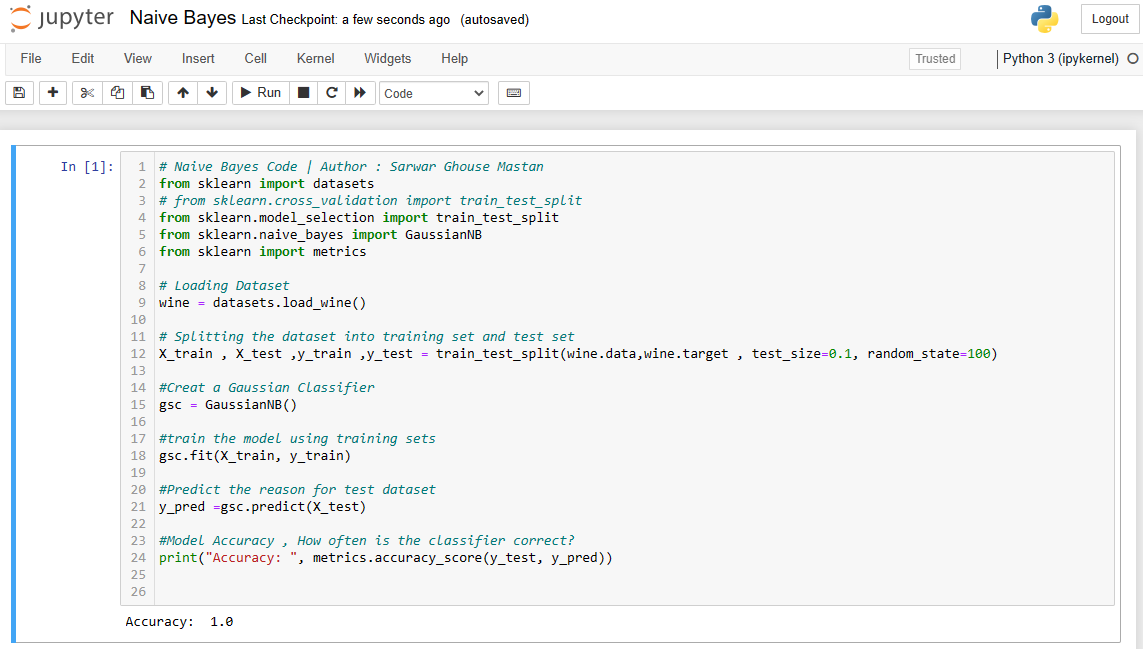### 25. Explain K-means Clustering and the steps for achieving K-means Clustering.

Ans: K-means (Macqueen, 1967) is among the most basic unsupervised learning methods for dealing with the well-known clustering challenge. K-means clustering is a signal processing-derived vector quantization method that is commonly utilized in data mining clustering algorithms.

The K-means algorithm can be applied as below if k is given:

• Creating k subsets (non-vacant) by dividing the objects.
• Finding the centroids of the current division.
• Allocating every point to a certain cluster.
• Determine the separations among each point, then allot points to the cluster that is closest to the centroid.
• Finding the newly formed cluster's core after reallocating the points.

### 26. In the K-means algorithm what does "means" signify?

Ans: The 'means' signifies data averaging or determining the centroid.

There are k-medoids and k-medians algorithms as well.

K-medoids reduce the total of dissimilarities among points tagged as belonging to a cluster as well as a point defined as the cluster's center. k-medoids select data points as centers (exemplars or medoids) unlike the K-means algorithms.

A variation of the K-means clustering is K-medians. In it, each cluster's centroid is determined by calculating the median rather than the mean.

### 27. How can I determine 'K-means' ideal amount of clusters? Describe the elbow technique and the elbow curve.

Ans: The key premise underlying partitioning methods like K-means clustering is constructing clusters in a way that the total WSS (within-cluster sum of a square) [or total intra-cluster variation] is minimal. The closeness of the clustering is calculated by the total WSS and should be as low as possible.

The Elbow technique calculates total WSS as a function of cluster count: One should select a variety of clusters such that adding an additional cluster does not significantly increase the total WSS.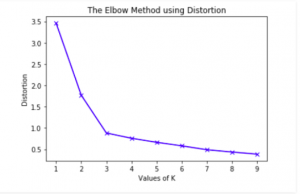Notice the elbow at k =3.

The ideal number of clusters are as follows:

• Calculate the clustering algorithm (K-means clustering, for example) for various K values. Let's say, changing K's value from 10 to 100 clusters.
• Calculate the addition of squares in the cluster, WSS, for each value of K.
• Plot the WSS curve based on the number of clusters K.
• The position of the knee (bend) in the graph is frequently used to determine the number of clusters.

### 28. What is the difference between K-means and Hierarchical Clustering?

Ans: K-means Clustering and Hierarchical Clustering work well together. The researcher is uninformed of the number of clusters to be created in hierarchical Clustering, but how many clusters are to be created in K-means Clustering is stated beforehand.

Advice- If you don't know how many clusters to build, apply hierarchical Clustering to figure it out. Then you may use K-means Clustering to create more suitable clusters. Hierarchical Clustering is just a one-time process whereas K-means is an iterative method.

### 29. What are the advantages and disadvantages of using K-means Algorithms?

Ans:

• If the variables are large and k is kept modest, K-means are usually computationally quicker than hierarchical clustering.
• When the clusters are round, K-means generates more compact clusters in comparison to hierarchical clustering.

• The value of K is difficult to anticipate.
• It doesn't perform properly when the cluster is global.
• Different beginning partitions might lead to various end clusters.
• Difficult to deal with distinct volume and size clusters (in the real sample).

K-means code snippet: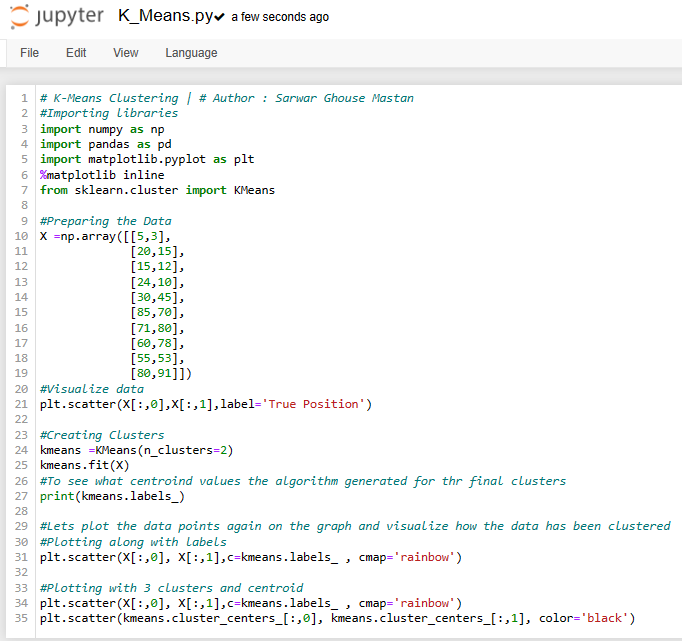### 30. What does Hierarchical Clustering mean?

Ans: A type of unsupervised learning approach used to combine unlabeled data points with comparable features is hierarchical clustering. There are two categories for hierarchical clustering techniques.

In hierarchical agglomerative algorithms, each piece of data is regarded as a distinct cluster, and the pairings of clusters are subsequently progressively agglomerated (bottom-up technique) or amalgamated.

Hierarchical dividing algorithms in divisive hierarchical algorithms, on the other hand, all data points are viewed as one huge cluster, and the clustering process entails splitting (Top-down method) the one big cluster into several tiny clusters.

### 31. What is the Procedure for Performing Agglomerative Hierarchical Clustering?

Ans: The most used and important Hierarchical clustering, i.e., agglomerative. Below are the steps-

• Step 1 − Assess every point of information as a segregated cluster. As a result, we'll have, let's say, K clusters at first. The initial data points also will be K.
• Step 2 − In this step, we will create a large cluster by connecting two nearby data points. This yields K-1 clusters.
• Step 3 − Now, by linking 2 closet clusters we can construct additional clusters. We'll have a total of K-2 clusters.
• Step 4 − To build a single large cluster, repeat the preceding three stages until K equals zero, i.e., there are no more data points to connect.
• Step 5 − Finally, after creating a single large cluster, dendrograms will be utilized to split the issue into numerous clusters.

### 32. What is Dendrogram and what is its importance in Hierarchical Clustering?

Ans: A dendrogram is a Tree Diagram that displays hierarchical grouping-linkages between comparable groups of data. They are commonly used in biology to demonstrate the clustering of genes or samples, although they may represent any form of clustered data.

Once the large cluster is created, the dendrogram's duty begins. Depending on the situation, a dendrogram would be employed to divide the clusters into various clusters of similar data points.

Parts of Dendrogram: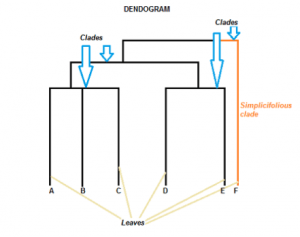Hierarchical Clustering Code Snippet: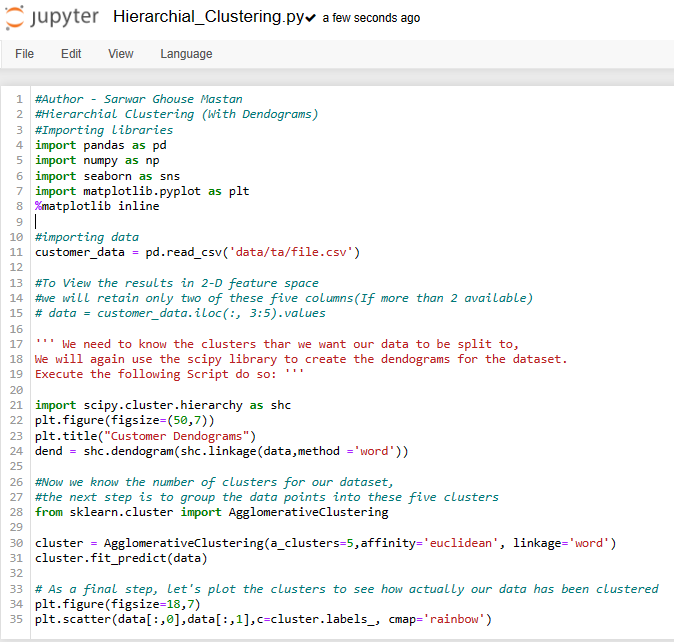### 33. What is Boosting?

Ans: Boosting is a technique for turning inferior learners into superiors. Each new tree in boosting fits an improved version of the initial data set.

Purpose of Boosting: It helps the weak learner be modified to improve.

How it evolved: AdaBoost, or Adaptive Boosting, was the first Boosting algorithm that gained popularity. Further, it evolved and generalized as Gradient Boosting.

Ans: Adaboost creates one strong learner by transforming a group of weak learners. The decision trees consisting of a single division, known as decision stumps, are weak learners. When AdaBoost generates its initial decision stump, all observations are equally weighted. The wrongly categorized observations now have greater weight than correctly classified ones, which helps in remedying the preceding issue. AdaBoost techniques apply to both classification and regression applications.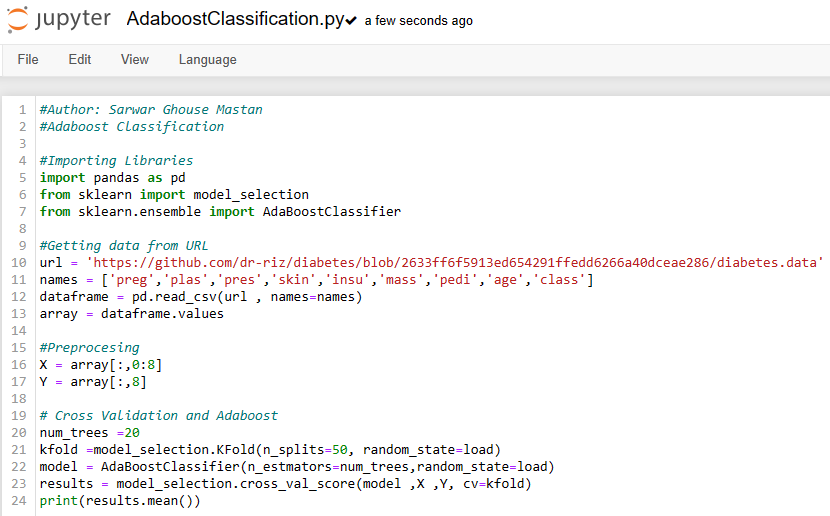### 35. What is Gradient Boosting Method (GBM)?

Ans: Gradient Boosting occurs when in an ensemble you keep adding predictors one at a time, each one correcting the one before it. However, unlike AdaBoost, which changes the weights for each incorrectly categorized observation at each iteration, the Gradient Boosting approach attempts to match the new predictor to the previous predictor's residual mistakes.

Gradient Descent is applied to identify flaws in the last learner's predictions by GBM. The GBM algorithm can be given in the following steps.

F1(x) = y to match a model with the information.

Make a new model with F2(x) = F1(x) + h1(x).

By consistently adding weak learners, we can account for a large portion of the mistake in the original model and minimize it over time.

### 36. What is XGBoost?

Ans: XGBoost is known as eXtreme Gradient Boosting. It is a decision tree solution that is gradient-boosted and tailored for performance and speed. Gradient boosting machines are notoriously slow to construct due to sequential model training. These really aren't particularly scalable. Hence, XGBoost is focused on model performance and computational speed. XGBoost provides

• During training, you may parallelize tree construction by employing your CPU cores.
• Distributed computing trains huge models on a cluster of devices.
• Out-of-Core Computing is used for exceedingly big datasets that cannot be stored in memory.
• Data structure and algorithm cache optimization to maximize hardware utilization.

XGBoost Code Snippet: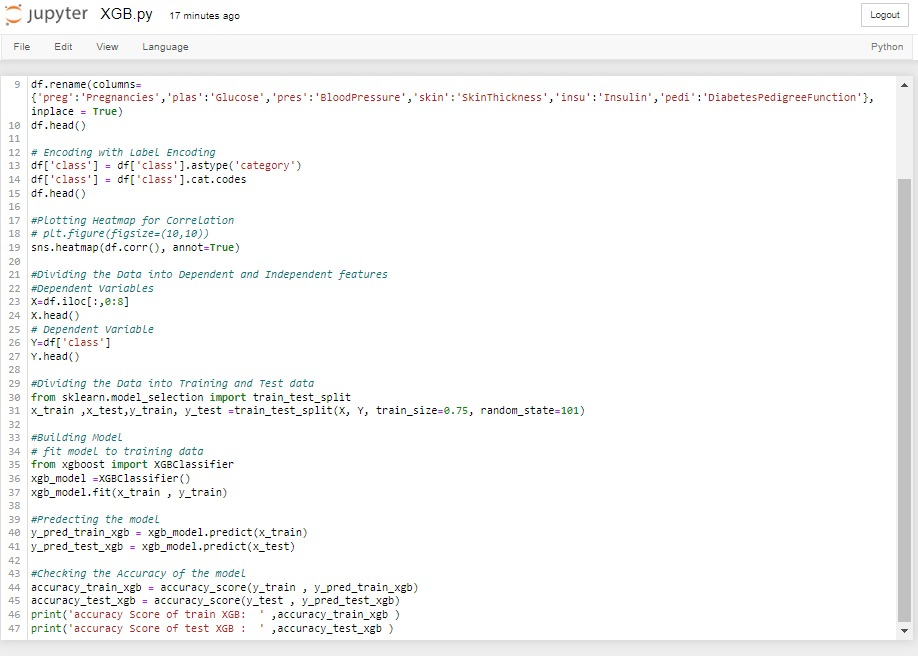### 37. What are the basic enhancements to Gradient Boosting?

Ans: Gradient boosting seems to be a strategy that rapidly overfits a trained model because it is greedy. It can profit from regularisation approaches that persecute different system sections and enhance overall algorithm performance by eliminating overfitting.

Take a look at these 4 improvements to simple gradient boosting:

1. Penalized Learning
2. Tree Constraints
3. Random sampling
4. Shrinkage

Tree Constraints: A nice general heuristic is that the greater constricted the single trees are, the more trees you'll require in the model; conversely, the less constricted the single trees are, the lesser trees you'll require.

Some limits that can be imposed on decision tree building are as follows:

• Overfitting may be exceedingly sluggish when increasing the tree numbers in the model. The recommendation is to maintain adding trees until no more improvement is shown.
• Deeper trees are more complicated, whereas shorter trees are desired. In general, 4-8 levels produce better outcomes.
• The number of leaves or nodes, such as depth, can limit the tree size but does not limit it to a symmetrical form if additional restrictions are utilized.
• The quantity of observations for each split establishes a minimal restriction on the training data number available at a training node prior to considering a split.
• The minimal improvement to lose is a constraint on the efficiency of every split introduced to a tree.
1. Penalized Gradient Boosting: The parametric trees and their design can be subjected to additional limitations. As weak learners, traditional decision trees such as CART are not utilized. Instead, a regression tree is utilized, which has numerical values inside the leaf nodes (also known as terminal nodes). In some literature, the values in the tree leaves are referred to as weights. L1 and L2 weights regularisation may be used to regularize the trees' leaf weight values. The additional regularization factor softens the complete learning values to prevent over-fitting. Intuitively, the regularised goal will favor models with simple and predictive functions.
2. Weighted Updates: Each tree's predictions are combined together successively. Every tree's contribution is calculated to the sum which slows down the algorithm's learning. This weighting is referred to as a learning rate or a shrinkage.
3. Stochastic Gradient Boosting: Enabling trees to really be greedily constructed from subsets of said training dataset was a significant breakthrough in bagging groups and the random forest. In gradient-boosting models, the same advantage may be employed to lower the connection between the trees inside the sequence. This type of boosting is known as stochastic gradient boosting. A randomized subsample of said training data (without replacing) is obtained from the entire training dataset at each iteration. The randomly chosen subsample is then utilized to adapt the base learner rather than the entire sample.

### 38. What is Dimensionality Reduction? Why is it used?

Ans: The process of transforming a dataset. That data must be transformed from data with large dimensions to data with smaller dimensions. It must also guarantee that comparable information is conveyed concisely.

Despite the fact that we employ these strategies to handle Machine Learning challenges, the challenge is to improve the regression or classification task's characteristics.

### 39. What are the commonly used Dimensionality Reduction Techniques?

Ans: Dimensionality reduction approaches include the following:

• Linear Discriminant Analysis (LDA)
• Principal Component Analysis (PCA)
• Generalized Discriminant Analysis (GDA)

### 40. How does PCA work? When to apply it?

Ans:
Principal Component Analysis (PCA) is a non-parametric, unstructured quantitative tool that is largely employed in ML to reduce dimensionality.

A high-dimensional dataset has a significant amount of features. In the Machine Learning area, the fundamental issue associated with large dimensionality is model overfitting, which lowers the capacity to generalize beyond the instances in the training set.

PCA in Layman's Term: Consider the 2D XY plane.

Let's think of variances like the information scatter, or the separation between the two distant locations, for the purpose of intuition.

Assumption:

In general, it is thought that datasets with a wide variation offer greater information than those with a slight variation. (This might be true or false.) This is the presumption that PCA wants to take advantages.

I give you 4 points – {(1,1), (2,2), (3,3), (4,4)}

(all lie on the line X=Y)

What is the variance on X-axis?

Variance(X) = 4-1 = 3

What is the variance on Y-axis?

Variance(Y) = 4-1 = 3

Can we somehow get new data with a bigger variance?

Rotate your XY system by 45 degrees anticlockwise. What happens? The line X=Y has now become the X(new)-axis. And, X = -Y is now the Y(new)-axis. Let's compute the variance again (in the form of distance)

Variance(X(new)) = distance ((4,4), (1,1)) = sqrt(18) = 4.24

Variance(Y(new)) = involves a computation.

### 41. What benefit did such rotation provide for us?

Ans: Original data had the highest variance on any axis as 3. This rotation gave us a variance of 4.24

That was a brief description of how PCA works. For the sake of completeness,

Eigenvalues are the variances of data along a certain axis inside the new coordinate frame. Eigenvalue(X(new)) = 4.24.

Eigenvectors = the vectors that serve as the new coordinate program's representation. From previous sample, vector [1,1]= eigenvector for X(new), [1,-1]= eigenvector for Y(new). Since they are just directions – solvers typically give us unit vectors.

Data transformation

After obtaining the eigenvectors, one may create a new position inside the current coordinate by doing a dot product of the eigenvector and the initial position..

Steps of PCA:

1. Determine the covariance matrix X of the data points.
2. Calculate eigenvectors and corresponding eigenvalues.
3. Sort eigenvectors in decreasing order according to their provided value.
4. Initial k eigenvectors = The recent k dimensions.
5. Convert the original n-dimensional data points to k-dimensional data points.

PCA code snippet: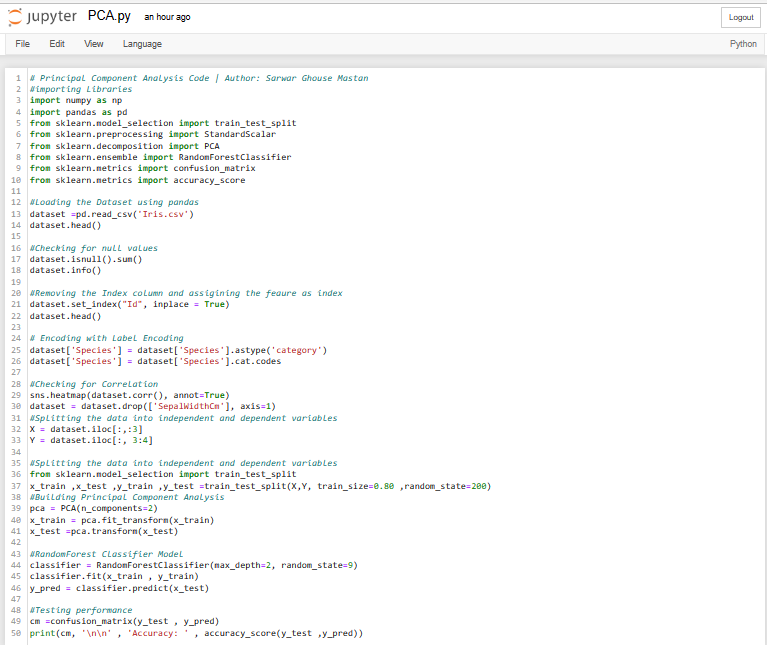### 42. How does LDA work? When to use it?

Ans: LDA is a technique for reducing 'dimensionality' while retaining as much class-discriminating information as feasible.

How does it work?

LDA assists you in determining the 'boundaries' of class clusters. It places your points on a plane, as much as possible separating your clusters, with every cluster possessing a relative (near) proximity to a centroid.

Dimensionality: Consider that you wish to divide a collection of information points into 2 dimensions into 2 categories. LDA reduces the dimensionality of your settings like so: K(Groups) = 2. 2-1 = 1.

But why so?

Because "at most K-1-dimensional affine subspace contains the K centroids."

What is the affine subspace?

The phrase "I'm going to generalize the affine qualities of Euclidean space" is expressed in a geometric notion or architecture.

Now, what are those affine properties of Euclidean space?

In three-dimensional space, a location could be represented by three coordinates. A location with two values in a two-dimensional sphere and a position with one value in a one-dimensional space ought to be capable to be represented. This two-dimensional problem's dimensionality was reduced via LDA to only one dimension. We may now begin the important task of responding to the information. We have two groups, as well as a line may connect any two places in either dimension.

Thus, a number of such data points are given to us, each with a 2D representation (x,y). These values will be divided into categories 1 or 2 using LDA.

### 43. Explain LDA steps?

Ans: LDA steps include:

1. For every class calculate its d-dimensional mean vector in the dataset.
2. Construct the dispersion matrix.
3. In a d * k directional matrix, select the k eigenvector having the highest eigenvalue by sorting the Eigen Vector into declining Eigen Value.
4. Through d * k eigenvector matrices, the data were projected into the resulting domain.

Multiplication of the matrix can be used to condense this.

Y = X * W, where X represents the n samples as n * d dimension matrices and W represents the modified n * k dimensional samples inside the current subspace.

LDA code snippet: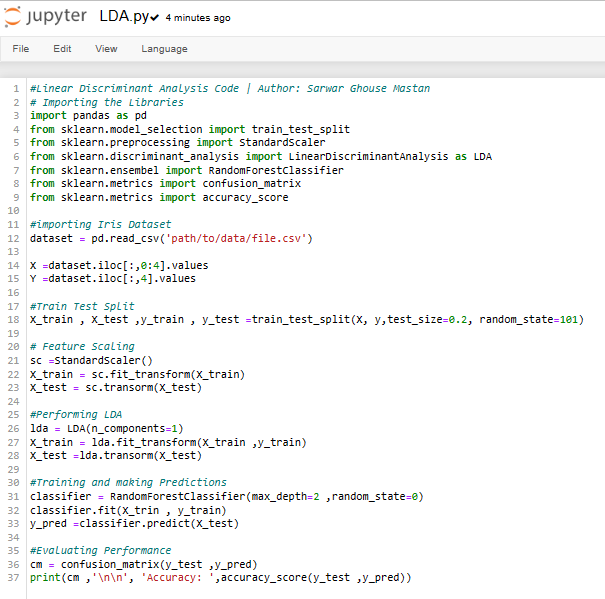### 44. What is GDA?

Ans: When we face a classification issue with constant random variables for input features, we may apply GDA, a generative learning technique that assumes p(x|y) is dispersed using a multivariate normal distribution and p(y) is dispersed as per Bernoulli.

GDA (Gaussian discriminant analysis) is a classification generative model in which each class distribution is treated as a multivariate Gaussian.

### 45. What pluses and minuses do Dimensionality Reduction offer?

Ans:

• Data compression is aided by dimension reduction, which results in little storage space.
• The calculation time is shortened.
• Additionally, it helps to get rid of any extraneous features.
• Dimensionality reduction aids in data compression and storage space reduction.
• It reduces the time needed to execute the same computations.
• There is less computation when there are fewer dimensions. Dimensions can also enable the use of unsuitable methods for a high number of dimensions.
• It handles multicollinearity, which enhances model performance. It gets rid of unnecessary features. For instance, it serves no purpose to hold a value in two different measurement types (in and m).
• Data reduction to 2D or 3D dimensions may allow us to plot and display it more precisely. Patterns can thus be seen more vividly.

• It may result in some data loss.
• However, PCA finds linear relationships between variables, which is not always desired.
• Furthermore, PCA fails to characterize datasets when covariance and mean are inadequate.
• In addition, we may not know how many main components to keep-in practice, broad rules are followed.

### 46. What is Time series?

Ans: A time series is a series of numerical data points presented in ascending order. It follows the movement of the selected data points over a predetermined time period and captures the datasets at regular intervals. There aren't any minimum or maximum time requirements for time series. Analysts frequently use time series to conduct analysis based on their individual needs.

Time Series Use Cases:

• Retail demand forecasting, sourcing, and dynamic pricing
• Used in Healthcare to forecast pandemic spread, diagnostics, and prescription planning
• The price projection for customer-centric apps, as well as improved user experience

### 47. What is a Box-Cox transformation?

Ans: A Box-Cox transformation converts non-normal dependent variables into normal shapes. Normality is a key assumption for several statistical approaches; if your data isn't normal, using a Box-Cox means you can perform more tests.

### 48. What role does maximum likelihood play in logistic regression?

Ans: The maximum likelihood equation aids in estimating the most likely values of the estimator's predictor variable coefficients, yielding the most probable results and relatively near to the true values.

### 49. Explain the chi-square test.

Ans: A chi-square test examines if a data sample set corresponds to a population. A chi-square test of independence examines whether two factors in a contingency table are connected.

A relatively tiny chi-square test score shows that the observed data fit the predicted data very well.

### 50. What exactly is the ROC curve?

Ans: ROC curve (receiver operating characteristics): The ROC curve depicts a binary classifier's diagnostic capabilities. It is estimated or constructed by plotting True Positive versus False Positive at various threshold values. AUC is the ROC curve's performance statistic (area under the curve). The model's predictive power increases with the size of the area beneath the arc.

## Summing Up:-

These are a few typical queries regarding machine learning interviews. Learning the fundamentals, different types of machine learning techniques, mathematics and heuristics components, and hands-on modeling to construct algorithms actually prepares you for an MNC machine learning interview. Choose to enroll in the Advanced AI and ML Certification to understand these machine learning interview questions deeply.